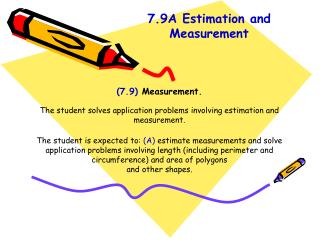Download Presentation(7.9) Measurement.(7.9) Measurement. - PowerPoint PPT Presentation

Download Presentation(7.9) Measurement.
An Image/Link below is provided (as is) to download presentation

Download Policy: Content on the Website is provided to you AS IS for your information and personal use and may not be sold / licensed / shared on other websites without getting consent from its author. While downloading, if for some reason you are not able to download a presentation, the publisher may have deleted the file from their server.

- - - - - - - - - - - - - - - - - - - - - - - - - - - E N D - - - - - - - - - - - - - - - - - - - - - - - - - - -
Presentation Transcript

1. 7.9A Estimation and Measurement (7.9) Measurement. The student solves application problems involving estimation and measurement. The student is expected to: (A) estimate measurements and solve application problems involving length (including perimeter and circumference) and area of polygons and other shapes.

2. The Grade 7 Mathematics Chart lists formulas for circumference and area of circles. • When using a formula to solve a problem, follow these steps: • Identify the formula that applies to the problem you are solving. • Identify what the variables in the formula stand for. • Substitute the variables in the formula with their values from the problem. • Perform the calculations. Remember to use the correct order of operations. • State the solution to the problem using the appropriate units of measurement.

3. Estimation and Measurement The answer to any measurement problem can be estimated before finding the exact answer by rounding the numbers in the problem before working it out. The estimate tells approximately what the answer will be. If estimation is done first, a decision can be made whether the actual calculated answer is reasonable. Estimation is used when actually solving some measurement problems. For example, problems that ask about how many, approximately how long, or whether a certain number is a reasonable answer to a problem. Estimation is actually required to solve these types of problems.

4. Another type of estimation involves pi, or π. For all circles, the ratio of the circumference to the diameter (C/d) is always the same. This ratio is called pi (π). The value of π on the Grade 7 Mathematics Chart is given as 3.14 or . That means the circumference of a circle is a little more than three times as long as its diameter. These numbers are only estimates of the value of π. The actual value of π is a number that begins 3.1415926… and continues forever without repeating a pattern. When a formula contains π, the answer is not exact if π is replaced with an approximated value. If π is not replaced and remains as part of the answer, then the answer is exact. 22 7